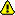# CAGMon - A Detchar Tool for Noise Propagation using Correlation Analysis

## Project Goal

The goal of this project is to find a systematic way of identifying the abnormal glitches in the gravitational-wave data using various methods of correlation analysis. Usually the community such as LIGO and Virgo uses a conventional way of finding glitches in auxiliary channels of the detector - Klein-Welle, Omicron, Ordered Veto Lists, etc. However, some different ways can be possible to find and monitor them in a (quasi-) realtime. Also the method can point out which channel is responsible for the found glitch. In this project, we study its possibility to apply three different correlation methods - maximal information coefficient, Pearson's correlation coefficient, and Kendall's tau coefficient - in the gravitational wave data from LIGO detector.

## Participants

• John J. Oh (lead, NIMS)
• Young-Min Kim (UNIST)
• More....

## Preliminaries

### Methods

#### Pearson's Correlation Coefficient

• PCC is a measure of a linear correlation between two random variables.
• Pearson's r is defined as:
• $r=\frac{\sum_{i=1}^{n} (x_i - \bar{x})(y_i-\bar{y})}{\sqrt{\sum_{i=1}^{n} (x_i-\bar{x})^2} \sqrt{\sum_{i=1}^{n} (y_i -\bar{y})^2}}$

#### Kendall's tau Coefficient

• $\tau = \frac{2(C-D)}{n(n-1)}$

where C and D are number of concordant and disconcordant pairs, respectively.

#### Maximal Information Coefficient

Basically, maximal information coefficient is defined using the mutual information score following the Ref. . Formally, the mutual information of two discrete random variables X and Y can be defined as: \begin{align} I(X;Y) = \sum_{y\in Y} \sum_{x\in X} p(x, y) \log \left(\frac{p(x, y)}{p(x)p(y)}\right) \end{align}

where p(x,y) is the joint probability distribution function of X and Y, and p(x) and p(y) are the marginal probability distribution functions of X and Y respectively. Intuitively, mutual information measures the information that X and Y share: it measures how much knowing one of these variables reduces uncertainty about the other. For example, if X and Y are independent, then knowing X does not give any information about Y and vice versa, so their mutual information is zero [Wikipedia].

It measures non-linear correlation between two data samples while the PCC (Pearson correlation coefficient) and the Spearman coefficient are only for the linear relationship.

With this definition of mutual information, MIC is defined by  \[ MIC(D) = \max_{xy

### Preliminary Knowledges

#### Previous Study Results

1. CAGMonLKR3 Guide : to be updated

2. GitLab: to be pushed

3. D. N. Reshef, Y. A. Reshef, H. K. Finucane, S. R. Grossman, G. McVean, P. J. Turnbaugh, E. S. Lander, M. Mitzenmacher, P. C. Sabeti, Science, 334, 1518 (2011).

1. python 3
2. numpy
3. scipy
4. matplotlib
5. minepy
6. gwpy

## Data & Code Preparation

### LIGO Data (2020.6~ )

• O3 glitches and their witness channels : https://wiki.ligo.org/DetChar/GlitchesandWitnesses

• list of glitches
• Magnetometer set
• Air Compressor set
• gpstimes: /home/cavaglia/karoo_omicron_O1aircompressor/data/gpstimes_O1aircompressor_sorted.txt @ LHO(ldas-pcdev1.ligo-wa.caltech.edu)

### Scheme & Goal## Observing Run Test of KAGRA O1

### Trigger-based Analysis (2020.6~ )

• Data: April 19 2020 SummaryPage

• Observing Information:
 Inspiral Range Plot Detector Sensitivity Omicron Glitch Gram• Loudest events by SNR:
• 10 loudest K1:CAL-CS_PROC_C00_STRAIN_DBL_DQ (Omicron) events by SNR with minimum 8s separation. Launch omega scans
 GPS time UTC time Duration Peak frequency Central freq. Bandwidth SNR GPS_start GPS_end Sam_Rate TStride FStride # of Samples Run Check 1271311593.609 April 19 2020 06:06:15.609 0.031 121.192 121.195 1.723 288.743 1271311586 1271311600 8192 2 7 16384/seg1271302217.998 April 19 2020 03:29:59.998 0.004 111.850 112.298 20.032 221.521 1271302210 1271302224 8192 2 7 16384/seg1271356741.438 April 19 2020 18:38:43.437 0.125 41.141 41.142 0.585 202.916 1271356734 1271356748 8192 2 7 16384/seg1271337318.002 April 19 2020 13:15:00.0 0.004 111.850 112.298 20.032 170.477 1271337311 1271337325 8192 2 7 16384/seg1271340918.001 April 19 2020 14:15:00.000 0.002 133.756 134.291 23.955 166.644 1271340911 1271340925 8192 2 7 16384/seg1271325225.998 April 19 2020 09:53:27.998 0.004 111.850 112.298 20.032 164.685 1271325220 1271325234 8192 2 7 16384/seg1271313018.002 April 19 2020 06:30:00.001 0.004 111.850 112.298 20.032 162.386 1271313011 1271313025 8192 2 7 16384/seg1271303583.984 April 19 2020 03:52:45.984 0.031 121.192 121.195 1.723 161.520 1271303576 1271303590 8192 2 7 16384/seg1271296947.422 April 19 2020 02:02:09.421 0.031 121.192 121.195 1.723 161.284 1271296940 1271296954 8192 2 7 16384/seg1271336417.998 April 19 2020 12:59:59.998 0.004 111.850 112.298 20.032 160.725 1271336410 1271336424 8192 2 7 16384/seg## Working Paper

• JKPS Special Issue: